﻿ A Discussion on the Practicability of an Analytical Method for Solving System of Linear Equations

### A Discussion on the Practicability of an Analytical Method for Solving System of Linear Equations

H. Saberi Najafi, S.A. Edalatpanah, N. VosoughiOPEN ACCESSPEER-REVIEWED

## A Discussion on the Practicability of an Analytical Method for Solving System of Linear Equations

H. Saberi Najafi1, S.A. Edalatpanah1,, N. Vosoughi1

1Department of Applied Mathematics, Lahijan Branch, Islamic Azad University, Lahijan, Iran

### Abstract

Unfailing real world problems in engineering, economics, finance, etc. can lead to solving a system of linear equations. In this paper we give a survey a new approach to solve this problem based on homotopy perturbation method. Furthermore, we show that the solving linear equations by using a new method called modified homotopy perturbation method, presented in [M.A. Noor, K.I. Noor, S. Khan, M. Waseem, Modified homotopy perturbation method for solving system of linear equations. Journal of the Association of Arab Universities for Basic and Applied Sciences., 13(2013)35-37] is impractical.

• Najafi, H. Saberi, S.A. Edalatpanah, and N. Vosoughi. "A Discussion on the Practicability of an Analytical Method for Solving System of Linear Equations." American Journal of Numerical Analysis 2.3 (2014): 76-78.
• Najafi, H. S. , Edalatpanah, S. , & Vosoughi, N. (2014). A Discussion on the Practicability of an Analytical Method for Solving System of Linear Equations. American Journal of Numerical Analysis, 2(3), 76-78.
• Najafi, H. Saberi, S.A. Edalatpanah, and N. Vosoughi. "A Discussion on the Practicability of an Analytical Method for Solving System of Linear Equations." American Journal of Numerical Analysis 2, no. 3 (2014): 76-78.

 Import into BibTeX Import into EndNote Import into RefMan Import into RefWorks

### 1. Introduction

Consider the following system of linear equations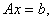(1.1)

where x denotes a vector in a finite-dimensional space and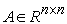.

Many of the problems that arise in technological, industrial and science situations are linear systems and there are some reliable methods for solving this class of problems ; see [1-12] and the references therein.

Here, we study alternative approach to solve (1.1) based on Homotopy Perturbation Method (HPM). The homotopy perturbation method, first proposed by the Chinese mathematician in  and was further improved and developed for solving a wide class of problems arising in various branches of pure and applied sciences; see [14, 15, 16, 17, 18] and the references therein.

Recently, some researches applied analytical methods for solving linear equations. Karamati , based on HPM proposed a new algorithm for linear equations. Liu  applied HPM to solve the linear systems and derive conditions to check the convergence of the homotopy series. Saberi Najafi and Edalatpanah  presented an analytical attitude for solving linear systems, based on Homotopy Analysis method (HAM) and analyzed the convergence properties of the proposed method. Very recently, Noor et al., in  used the modified homotopy perturbation method to solving the system of linear equations. In this paper, we study HPM models to solve (1.1). Furthermore, we show that the Noor et al.'s method is impractical.

### 2. HPM Models for Solving System of Linear Equations

A homotopy between two continuous functions f and g from a topological space X to a topological space Y is defined to be a continuous function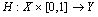from the product of the space X with the unit interval [0, 1] to Y such that, for all points x in X, H(x; 0) = f(x) and H(x; 1) = g(x). If we think of the second parameter of H as "time", then H describes a "continuous deformation" of f into g. At time t = 0, we have the function f and at time t = 1 we have the function g.

Next, we study some, numerical models for solving linear systems based on HPM.

2.1. Karamati's Model

Consider the linear equation(1.1),where;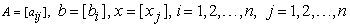Let;(2.1)

where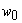is a known vector.

Then we define homotopyas follows: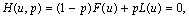(2.2)

whereis an embedding parameter.

Obviously, we will have,(2.3)(2.4)

According to the HPM, we can first use the embedding parameter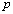as a small parameter, and assume that the solution of Eq.(1.1) can be written as a power series in: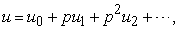(2.5)

and the exact solution is obtained as follows:(2.6)

Putting Eq.(2.5) into Eq.(2.2),and comparing the coefficients of identical degrees of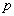on both side, we findAnd in general,Taking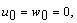yields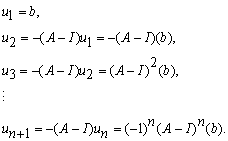Therefore the solution can be of the form(2.7)

The convergence of the series (2.7) is proved for diagonally dominant matrix A; see .

2.2. Liu's Model

Karamati's model is efficient for solving system of linear equations. However, this model does not converge for some systems when the spectral radius is greater than one.

To make the improved model, Liu in  added the auxiliary parameter and the auxiliary matrix to the HPM. This method is as follows;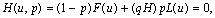(2.8)

where,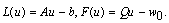(2.9)

Here,is an auxiliary parameter,is the auxiliary matrix and the operatoris decided by the splitting matrix.Then his iterative scheme foris;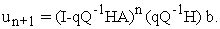(2.10)

Therefore;(2.11)

And finally by setting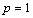, the obtained solution is as follows,(2.12)

The author shows that the homotopy series converges much more rapidly than the direct methods. Moreover, he has adapted the Richardson, Jacobi and the Gauss–Seidel methods to choose the splitting matrix and obtained that the homotopy series converged rapidly for a large sparse system with a small spectral radius.

Now, we study another model and show that this model is impractical.

2.3. Noor et al.'s Model (Modified HPM to Solve System of Linear Equation)

Noor et al.  forand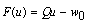, where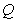is any splitting matrix andis a known vector, defined homotopy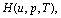as follows: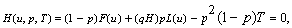(2.13)

where,is an embedding parameter andis non-zero auxiliary parameter,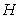is the auxiliary matrix and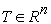is an arbitrary operator.

Following the above procedures and some algebra; (see ), the exact solution of problem (1.1) is presented to be as;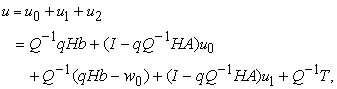(2.14)

where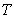has be obtained from ;(2.15)

Therefore,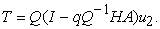(2.16)

By substitution (2.16) in (2.14), we have;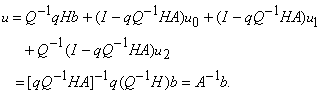(2.17)

Shortly, the main result in the above model is as follows:

The exact solution of linear system (1.1) is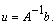As we know, this is trivial. On the other hand, based on Eq. (2.17), the proposed method in  for solving (1.1) is as follows: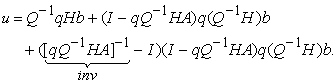(2.18)

i.e, Noor et al.'s model to solve Au=b, is (2.18). However,orin (2.18) are unknown. On the other hand, if we know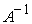, then any methods for linear systems are redundant. Therefore, the mentioned method is impractical.

### 3. Conclusions

In this paper, three numerical models based on homotopy perturbation methods (HPM) are studied for solving the system of linear equations. Furthermore, we show that one of these models is impractical.

### References

  D. M. Young, Iterative solution of large linear systems, Academic Press, New York, 1971.In article  A. Berman, R. J. Plemmons, Nonnegative matrices in the mathematical sciences, Academic, New York, 1994.In article CrossRef  R.S. Varga, Matrix iterative analysis, second ed., Springer, Berlin, 2000.In article CrossRef  H. Saberi Najafi, S. A. Edalatpanah, Comparison analysis for improving preconditioned SOR-type iterative Method, Numerical Analysis and Applications., 6(2013) 62-70.In article CrossRef  H. Saberi Najafi, S. A. Edalatpanah, On the Convergence Regions of Generalized AOR Methods for Linear Complementarity Problems. J. Optim. Theory. Appl., 156(2013) 859-866.In article CrossRef  H. Saberi Najafi, S. A. Edalatpanah, On the Application of Liao's Method for Solving Linear Systems. Ain Shams Engineering Journal, 4 (2013) 501-505.In article CrossRef  H. Saberi Najafi, S. A. Edalatpanah, A Note on “A new method for solving fully fuzzy linear programming problems”. Appl. Math. Model., 37 (2013) 7865-7867.In article CrossRef  H. Saberi Najafi, S. A. Edalatpanah, On the Application of Liao's Method for Solving Linear Systems. Ain Shams Engineering Journal, 4 (2013) 501-505.In article CrossRef  H. Saberi Najafi, S. A. Edalatpanah, A.H.Refahi Sheikhani, A new model of (I+S)-type preconditioner for system of linear equations. Journal of Mathematical Modeling, 1(2013) 1-14.In article  H. Saberi Najafi, S. A. Edalatpanah, G.A. Gravvanis, An Efficient Method for Computing the Inverse of Arrowhead Matrices. Applied Mathematics Letters, 33 (2014) 1-5.In article CrossRef  H. Saberi Najafi, S. A. Edalatpanah, on the modified symmetric successive overrelaxation method for augmented systems. Computational and Applied Mathematics,(2014).In article  H. Saberi Najafi, S. A. Edalatpanah, A.H.Refahi Sheikhani, Convergence Analysis of Modified Iterative Methods to Solve Linear Systems. Mediterranean Journal of Mathematics, (2014).In article  J.H. He, Homotopy perturbation technique. Computational Methods in Applied Mechanics and Engineering, 178(1999), 257-262.In article CrossRef  J.H. He, Application of HPM to nonlinear wave equations. Chaos, Solitons and Fractals. 26(2005) 695-700.In article CrossRef  M. Dehghan, F. Shakeri, Use of He's homotopy perturbation method for solving a partial differential equation arising in modeling of flow in porous media, Journal of Porous Media.,11(2008)765-778.In article CrossRef  J. Saberi-Nadjafi,A. Ghorbani, He’s homotopy perturbation method: An effective tool for solving nonlinear integral and integro-differential equations, Computers & Mathematics with Applications, 58(2009) 2379-2390.In article CrossRef  F. Soltanian, M. Dehghan, S.M. Karbassi, Solution of the differential algebraic equations via homotopy perturbation method and their engineering applications. International Journal of Computer Mathematics. 87(2010)1950-1974.In article CrossRef  H. Saberi Najafi, S. A. Edalatpanah, Homotopy Perturbation Method for Linear Programming Problems. Appl. Math. Model. 38(2014), 1607-1611.In article CrossRef  B. Karamati, An approach to the solution of linear system of equations by He’s homotopy perturbation method, Chaos. Solitons and Fractals., 41(2009)152-156.In article CrossRef  H.K. Liu, Application of homotopy perturbation methods for solving systems of linear equations. Applied Mathematics and Computation., 61(2011) 2555-25561.In article CrossRef  M.A. Noor, K.I. Noor, S. Khan, M. Waseem, Modified homotopy perturbation method for solving system of linear equations. Journal of the Association of Arab Universities for Basic and Applied Sciences. 13(2013)35-37.In article CrossRef